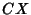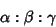## Isogonal ConjugateThe isogonal conjugateof a pointin the plane of the Triangleis constructed by reflecting the lines,, andabout the Angle Bisectors at,, and. The three reflected lines Concur at the isogonal conjugate. The Trilinear Coordinates of the isogonal conjugate of the point with coordinatesareIsogonal conjugation maps the interior of a Triangle onto itself. This mapping transforms lines onto Conic Sections that Circumscribe the Triangle. The type of Conic Section is determined by whether the linemeets the Circumcircle,

1. Ifdoes not intersect, the isogonal transform is an Ellipse;

2. Ifis tangent to, the transform is a Parabola;

3. Ifcuts, the transform is a Hyperbola, which is a Rectangular Hyperbola if the line passes through the Circumcenter
(Casey 1893, Vandeghen 1965).

The isogonal conjugate of a point on the Circumcircle is a Point at Infinity (and conversely). The sides of the Pedal Triangle of a point are Perpendicular to the connectors of the corresponding Vertices with the isogonal conjugate. The isogonal conjugate of a set of points is the Locus of their isogonal conjugate points.

The product of Isotomic and isogonal conjugation is a Collineation which transforms the sides of a Triangle to themselves (Vandeghen 1965).

See also Antipedal Triangle, Collineation, Isogonal Line, Isotomic Conjugate Point, Line at Infinity, Symmedian Line

References

Casey, J. A Treatise on the Analytical Geometry of the Point, Line, Circle, and Conic Sections, Containing an Account of Its Most Recent Extensions with Numerous Examples, 2nd rev. enl. ed. Dublin: Hodges, Figgis, & Co., 1893.

Johnson, R. A. Modern Geometry: An Elementary Treatise on the Geometry of the Triangle and the Circle. Boston, MA: Houghton Mifflin, pp. 153-158, 1929.

Vandeghen, A. Some Remarks on the Isogonal and Cevian Transforms. Alignments of Remarkable Points of a Triangle.'' Amer. Math. Monthly 72, 1091-1094, 1965.